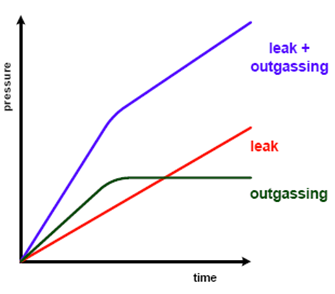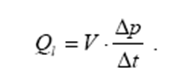## Pages

Showing posts with label pressure. Show all posts
Showing posts with label pressure. Show all posts

### Kinetic Theory of Gases - Important Points

1. The cohesive force (which binds molecules together) between particles of matter which constitutes a gas is extremely small.

2. Mathematical basis of Kinetic Theory of gases was established by Maxwell and Clausius.

3. Kinetic theory of gases relates macroscopic properties with microscopic properties of its molecules.

According to this theory,

4.  Molecules are rigid, perfectly elastic and identical in all respects.

5. The average distance traveled by a molecule between two collisions is known as Mean free path.

6.  On the basis of kinetic theory of gases, the pressure exerted by a gas is given by

P = (1/3)*(m*n*c²/)/V

where, 'n' is no. of moles, 'm' is mass of each molecule and 'M' is total mass of gas, V is volume of gas, c is average speed of molecules, c² is Mean square speed of molecules

c² = (c₁² + c₂² + .......+ cn²)/n

7.  Pressure exerted by a gas 'P'  is 2/3rd of total translational Kinetic energy of molecules per unit volume.

8. Mean kinetic energy of a molecule is

(1/2)mc² = (3/2)KT

where 'K' is Boltzmann Constant, K=R/N; R is universal gas constant & NA  is Avagadro's number.

9. Kinetic energy of a gram molecule of a gas = (3/2)RT

The number of atoms or molecules in a mole of substance is called Avogadro's number.
Its value is 6.023 x 10²³ mol⁻¹ .

11. Kinetic energy of a molecule depends upon absolute temperature 'T' and it is quite independent of its mass. This fact is known as Kinetic interpretation of temperature.

12. According to kinetic theory of gases, at absolute zero of temperature, the Kinetic Energy of gas becomes zero i.e. molecular motion ceases.

13. Above point is strictly not true because at T=0, the molecules do have some energy known as Zero Point energy.

14. Deduction of gas laws from kinetic theory:

i) Boyles law

P = (1/3)*Mc² ; PV=constant;

At constant temperature, pressure of a gas is inversely proportional to volume of a gas.

ii) Charles Law

PV ∝T ; The volume of gas at constant pressure is directly proportional to the temperature (or) pressure of a gas at consatnt volume is directly proportional to temperature.

N1=N2; Equal volume of ideal gases existing under same conditions of temperature and pressure contain equal number of molecules. This is called as Avogadro's law.

iv) Dalton's law or partial pressure

P=P1+P2+ ....

the total pressure exerted by gaseous mixture is sum of individual pressures that would be exerted if several gases occupied space in turn, alone.

v) Grahams law of diffusion

The rate of diffusion of a gas through a porous portion is inversely proportional to square root of its density.

Root mean square velocity (crms) = √c² = √(3KT)/m = √(3P)/ρ

15. Law of equipartition of energy (deduced by James Clark Maxwell)

i) Average value of the components of velocity 'C' (i.e. u,v & w) along 3 directions should be equal or for a molecule all 3 directions are equivalent i.e. u=v=w

ii) Total mean kinetic energy of molecule is E = (3/2)KT;

K is Boltzman constant and T is absolute temperature.

iii) A molecule has "three translational" degrees of freedom.

iv) Total Kinetic Energy of a dynamical system is equally divided among all its degree of freedom and it is equal or (1/2)KT per degree of freedom.This is called Law of eqipartition of energy.

v) For a "monoatomic molecule", we have only translational motion because they are not capable of rotation. Thus for one molecule of a monoatomic gas total energy E=(3/2)KT.

vi) For a "diatomic molecule" we can suppose it to be two sphere joined by a rigid rod. Such a molecule can rotate about any one of 3 mutually perpendicular axes. The rotational inertia about an axis along rigid rod is negligible compared to that about an axis perpendicular to rod, so rotational energy consists of to terms such as (1/2)I𝓌y² & (1/2)I𝓌z².

vii) For special description of center of mass of a diatomic gas molecule, 3 coordinates will be required. Thus, for a diatomic gas molecule having both rotational & translational motion;

E = (3/2)KT + 2(1/2)KT = (5/2) KT

viii) For "tri-atomic gases", each molecule contains 3 spheres joined together by rods so that molecule is capable of rotating energetically 3 mutually perpendicular axes. Hence, for triatomic molecule having both translational & rotational motion energy 'E' will be

E = 3(1/2)KT + 3(1/2)KT = 3KT

ix) If a molecule in all has 'f' degrees of freedom, its average total energy would be (1/2)fKT.

# Force definition

The push or pull of a body by an object is called force on the body.

or

Force is an external influence acting on a body to change its state of motion.

## Types of Forces

### Contact Force

Force which results when there is a direct physical contact between two interacting objects is known as contact force.

### Types of Contact Forces

Muscular Force
The force which we exert by using our muscles is called as muscular force.

Friction Force
The force which resists the motion of an object over the surface of other.  The direction of friction force is always opposite to the direction of the motion relative to the surface.

Normal Force
The friction force, on an object, which acts perpendicular to the surface of  its plane is called as Normal Force.

Tension
Tension is a pulling force acting on a object by means of a string against the gravitational force.

### Field Force

The force which occurs without any physical contact is known as force at a distance or field force.

Field is region of imaginary lines around an object. When an other body is placed in this region, it will experience the force. The greater the density of these imaginary lines, the stronger the forces in that region.

### Types of Field Forces

Magnetic Force
Magnetic force is a field force responsible for attraction of like poles and repulsion of unlike poles.

Electrostatic Force
Force exerted by a charged body on another charged body is known electrostatic force. This force is due to electric field from the charge.

Gravitational Force
The force of attraction that exists between any two masses n the universe is known as Gravitational force. This force is due to electric field from the charge. Gravitational force is also non contact force and hence it is also a field force.

## Net Force

Multiple forces can act on an object simultaneously at a time. When the forces act in same direction then net force will be addition of all forces. When the forces are in opposite direction, then net force will be difference of the forces. When the Net force is zero, there is no change in state of object.

The direction of motion of the object will be in the direction of Net Force. If the Net Force acts in direction of motion, the speed of the an object moving with constant speed also increase. If the Net Force acts in a direction opposite to the motion, then it either slows down the object or brings it to rest or it may change the direct of motion.

The unit of force in SI system is Newton(N).

## Pressure

The force acting perpendicularly on unit area of a surface is called as pressure.

Pressure = Force/Area

The unit of pressure in SI system is Newton/Meter² or N/m²

### WHAT IS LEAK RATE IN VACUUM SCIENCE?

Ideally it is impossible to build a vacuum system with out any leak. Without active pumping the pressure in a real system will rise with time.

Pressure rise is produced by outgassing and by gas molecules penetrating through leaks from the outside into the vacuum system.

The leak rate must be small enough not to prevent to reach the required pressure level.

A real leak will yield a linear pressure rate-of rise curve. The slope of the curve is a function of the leak rate and the volume of the system:Leak is related to how many molecules per unit time are being admitted into volume.
According to the gas laws, if volume, temperature and pressure are specified, it is possible to determine how many molecules are there inside the volume.

Torr-liter defines the molecules contained in a one liter volume at a pressure of 1 Torr at 0 °C.

22.4 liters of gas at 760 Torr and 0 °C equals 6.02x1023 molecules (Avogadro’s number)

1 Torr-liter would then contain about 3.5 x 1019 molecules.

std cc represents the number of molecules contained in a 1 cc volume at a pressure of 760 Torr and 0°C

1 std. cc contains about 2.7 x 1019 molecules

The leak rate is defined as the pV-throughput of a gas through a leak.
It is a function of the type of gas, pressure difference and temperature.

In a system of volume V the leakHere Δp is the pressure rise during the time interval Δt .